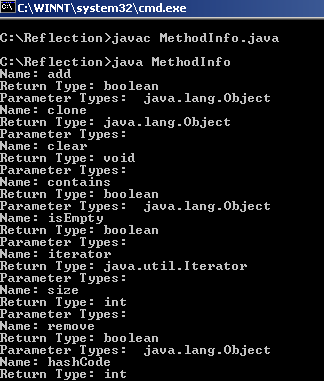Tutorials

# Getting Methods Information of a class

In this part of tutorial you will learn how to retrieve information of all methods of a class (that included in the program) by using the getMethods() method.

In this part of tutorial you will learn how to retrieve information of all methods of a class (that included in the program) by using the getMethods() method.

# Getting Methods Information of a class

In this part of tutorial you will learn how to retrieve information of all methods of a class (that included in the program) by using the getMethods() method. Here is an example that provides the usage of the getMethods() method in more detail.

Create an object of class. Now retrieve all methods list in Method[] array. Now we can get other information of that methods by using different methods on that method object.

Here is the example code of MethodInfo.java

MethodInfo.java

 ``` import java.lang.reflect.*; import java.util.HashSet; public class MethodInfo{   public static void main(String str[]){   HashSet set = new HashSet();   Class classObj = set.getClass();   Method[] methods = classObj.getMethods();   for (int i = 0; i < methods.length; i++) {  String methodName = methods[i].getName();   System.out.println("Name: " + methodName);   String returnString =  methods[i].getReturnType().getName();   System.out.println("Return Type: " + returnString);   Class[] parameterTypes =   methods[i].getParameterTypes();   System.out.print("Parameter Types: ");   for (int k = 0; k < parameterTypes.length; k ++) {  String parameterName =    parameterTypes[k].getName();  System.out.print(" " + parameterName);  }  System.out.println();   }  } }```

Output: